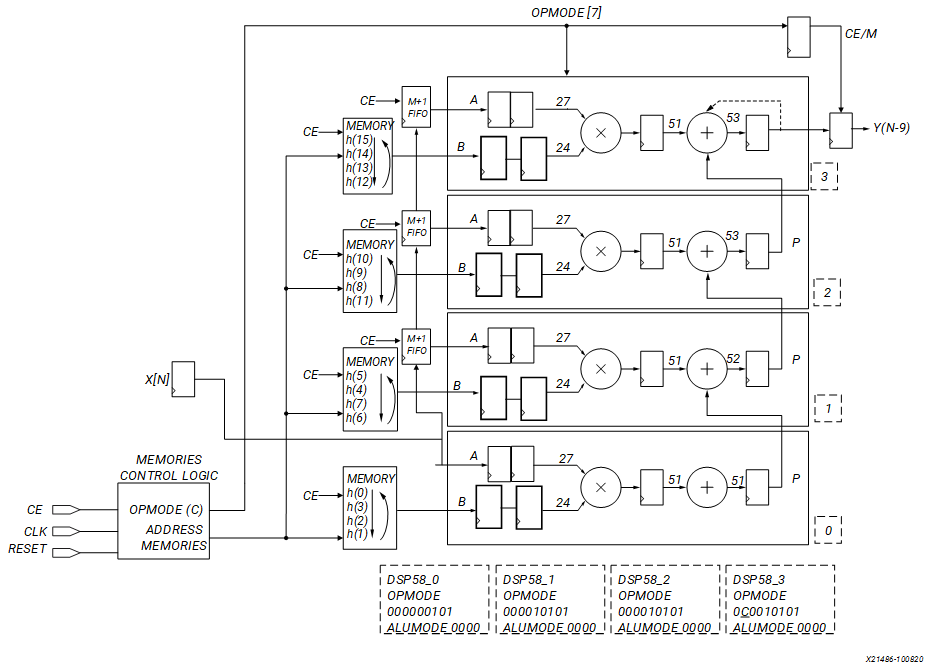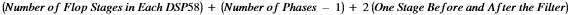# Decimating

## Versal ACAP DSP Engine Architecture Manual (AM004)

Document ID
AM004
Release Date
2022-09-11
Revision
1.2.1 English

Decimating is the process of reducing the number of samples representing a signal. Decimation is used in video applications like 4:4:4-to-4:2:2 conversions and HDTV-to-SDTV conversions. The following figure illustrates a 16-tap 4:1 decimator implemented using four parallel filters. The phases are related to the number of DSP58s and the number of coefficients is related to the memory depth (if RAM is used) or number of registers (SRL16). For every four-input sample, the decimator produces one output sample.

Figure 1. 16-Tap 1:4 DecimatorFor the 16-tap filter, the output sample (y) is the weighted average of 16 input samples (x) multiplied by 16 coefficients (h) as described in the following equation.

y[n] = (h0 × xn) + (h1 × xn – 1) + (h2 × xn – 2) + (h3 × xn – 3) + (h4 × xn – 4) + (h5 × xn – 5) + (h6 × xn – 6) + (h7 × xn – 7) + (h8 × xn – 8) + (h9 × xn – 9) + (h10 × xn – 10) + (h11 × xn – 11) + (h12 × xn – 12) + (h13 × xn – 13) + (h14 × xn – 14) + (h15 × xn – 15)

The input signals to each DSP58 are delayed by (M + 1) clocks from the previous DSP58. Shift registers are used to achieve this delay. An initial latency is given by the following equation.The 16-tap filter output is obtained at the fourth DSP58. The OPMODE must be changed dynamically to ensure functionality.

The reference design files associated with this use case are available in the multirate_FIR/polyphase_decimating_FIR directory in the design archive file, am004-versal-dsp-engine.zip.